# Thermodynamics Lecture Series First Law of Thermodynamics Energy

• Slides: 59Thermodynamics Lecture Series First Law of Thermodynamics & Energy Balance – Control Mass, Open System Applied Sciences Education Research Group (ASERG) Faculty of Applied Sciences Universiti Teknologi MARA email: [email protected] com http: //www 5. uitm. edu. my/faculties/fsg/drjj 1. htmlQuotesQuotes "One who learns by finding out has sevenfold the skill of the one who learned by being told. “ - Arthur Gutterman "The roots of education are bitter, but the fruit is sweet. " -AristotleResearch Findings – Retention % of Learning After 3 days period Read only – 10% See only 30% Hear only – 20% See + hear – 50% Say only – 70% Say & do simultaneously - 90%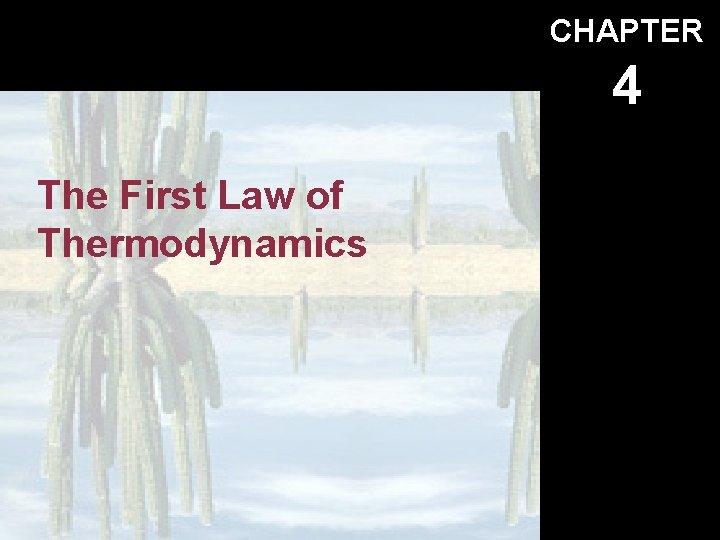CHAPTER 4 The First Law of ThermodynamicsIntroduction Objectives: 1. State the conservation of energy principle. 2. Write an energy balance for a general system undergoing any process. 3. Write the unit-mass basis and unit-time basis (or rate-form basis) energy balance for a general system undergoing any process. 4. Identify the energies causing the system to change.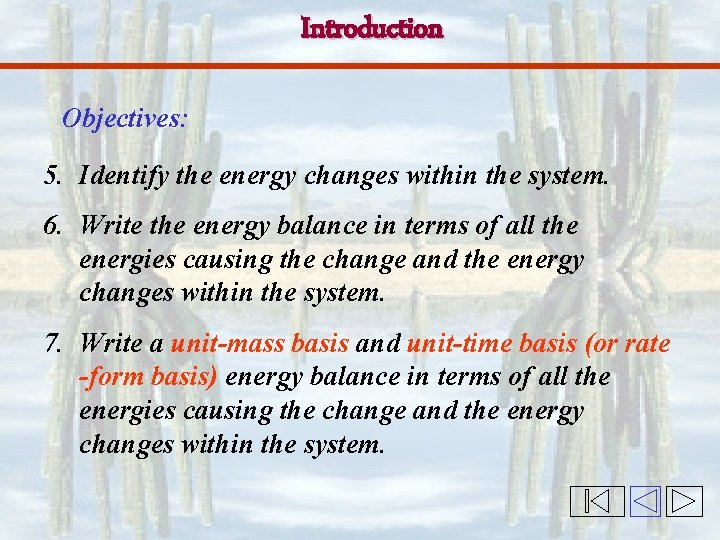Introduction Objectives: 5. Identify the energy changes within the system. 6. Write the energy balance in terms of all the energies causing the change and the energy changes within the system. 7. Write a unit-mass basis and unit-time basis (or rate -form basis) energy balance in terms of all the energies causing the change and the energy changes within the system.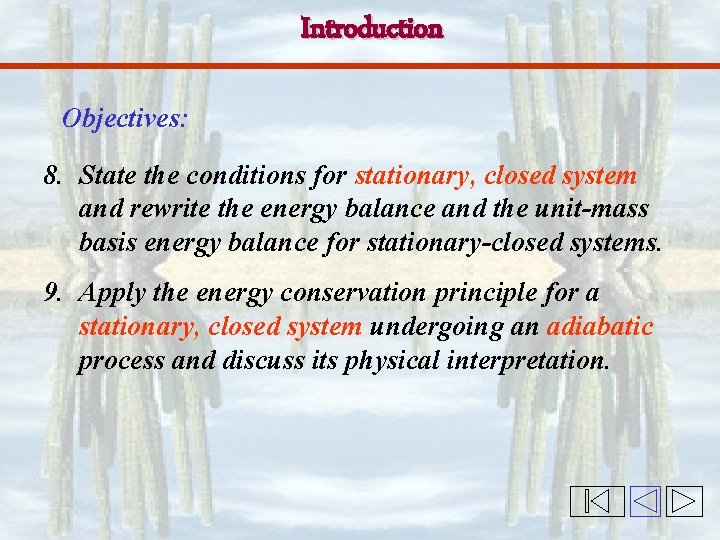Introduction Objectives: 8. State the conditions for stationary, closed system and rewrite the energy balance and the unit-mass basis energy balance for stationary-closed systems. 9. Apply the energy conservation principle for a stationary, closed system undergoing an adiabatic process and discuss its physical interpretation.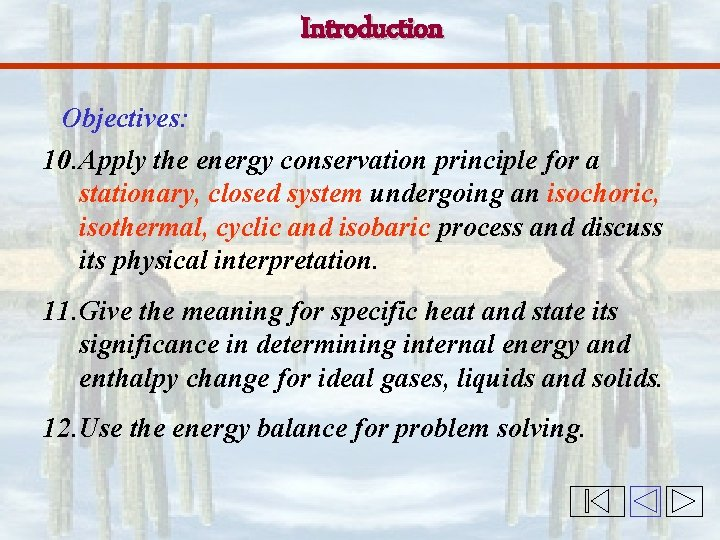Introduction Objectives: 10. Apply the energy conservation principle for a stationary, closed system undergoing an isochoric, isothermal, cyclic and isobaric process and discuss its physical interpretation. 11. Give the meaning for specific heat and state its significance in determining internal energy and enthalpy change for ideal gases, liquids and solids. 12. Use the energy balance for problem solving.Energy Transfer -Heat Transfer P = 100 k. Pa T = 99. 6 C SODA 5 C 3 -1 qin Nasi Lemak 20 C 200 C qout Sat. Vapor H 2 O: Sat. Liq. qin Oven Qin 25 C What happens to the properties of the system after the energy transfer?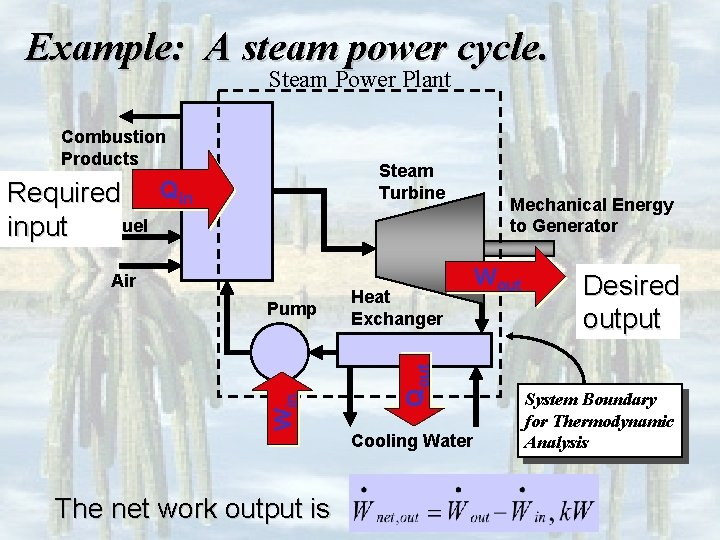Example: A steam power cycle. Steam Power Plant Combustion Products Steam Turbine Air Win Pump The net work output is Heat Exchanger Qout Required Qin Fuel input Cooling Water Mechanical Energy to Generator Wout Desired output System Boundary for Thermodynamic Analysis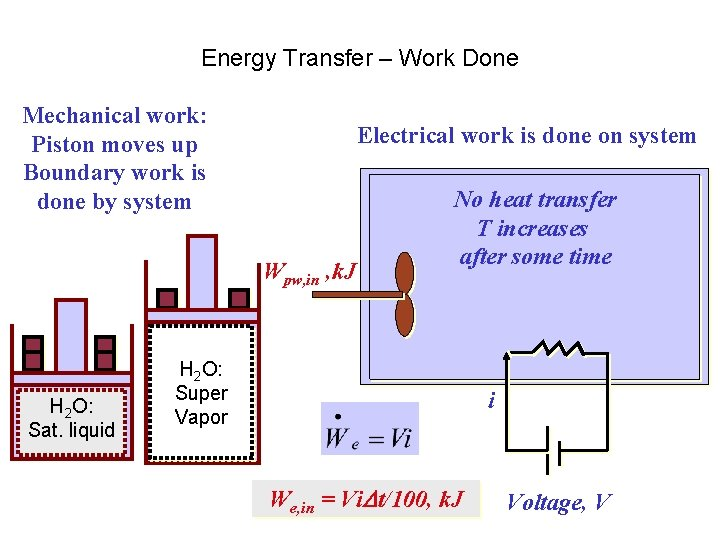Energy Transfer – Work Done Mechanical work: Piston moves up Boundary work is done by system Electrical work is done on system Wpw, in , k. J H 2 O: Sat. liquid No heat transfer T increases after some time H 2 O: Super Vapor i We, in = Vi t/100, k. J Voltage, VCopyright © The Mc. Graw-Hill Companies, Inc. Permission required for reproduction or display. FIGURE 4 -46 Pipe or duct flow may involve more than one form of work at the same time. 4 -8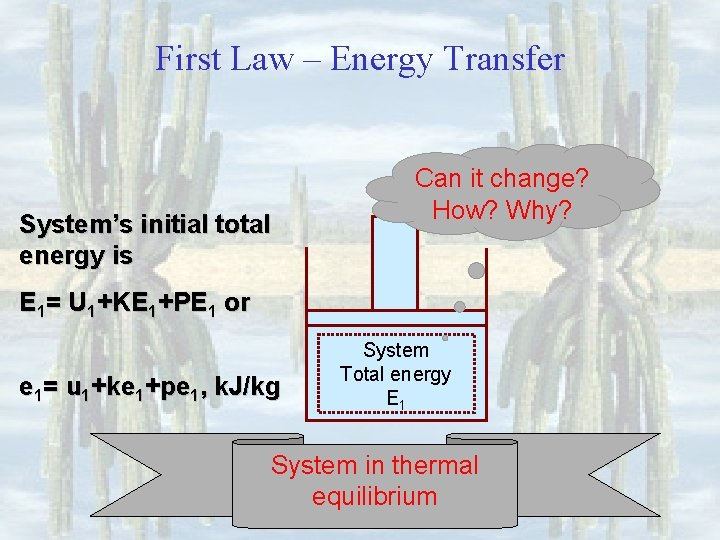First Law – Energy Transfer System’s initial total energy is Can it change? How? Why? E 1= U 1+KE 1+PE 1 or e 1= u 1+ke 1+pe 1, k. J/kg System Total energy E 1 System in thermal equilibriumFirst Law – Energy Transfer Movable boundary position gone up System expands E 1= U 1+KE 1+PE 1 System, E 1 System A change has taken place.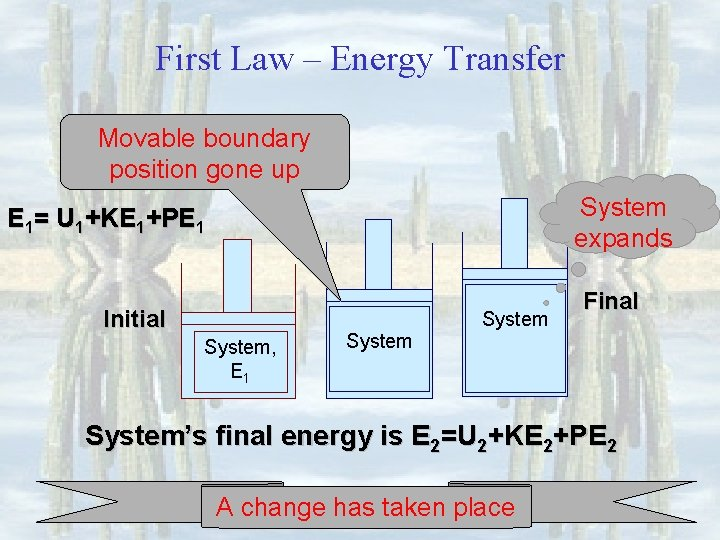First Law – Energy Transfer Movable boundary position gone up System expands E 1= U 1+KE 1+PE 1 Initial System, E 1 System Final System’s final energy is E 2=U 2+KE 2+PE 2 A change has taken place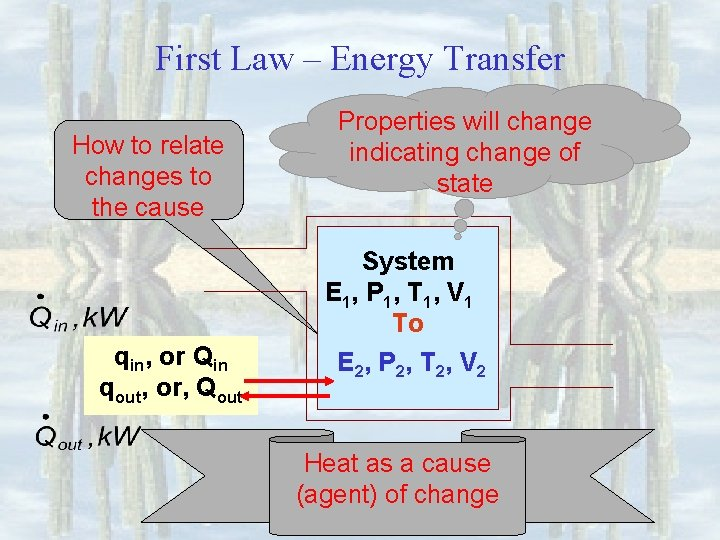First Law – Energy Transfer How to relate changes to the cause qin, or Qin qout, or, Qout Properties will change indicating change of state System E 1, P 1, T 1, V 1 To E 2, P 2, T 2, V 2 Heat as a cause (agent) of changeFirst Law – Energy Transfer How to relate changes to the cause Properties will change indicating change of state System E 1, P 1, T 1, V 1 To E 2, P 2, T 2, V 2 Work as a cause (agent) of change Win, k. J/kg Wout, in, k. J/kg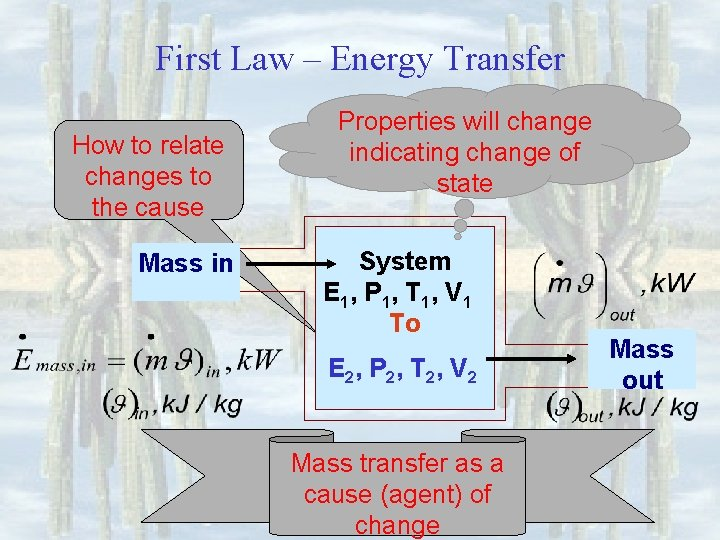First Law – Energy Transfer How to relate changes to the cause Mass in Properties will change indicating change of state System E 1, P 1, T 1, V 1 To E 2, P 2, T 2, V 2 Mass transfer as a cause (agent) of change Mass out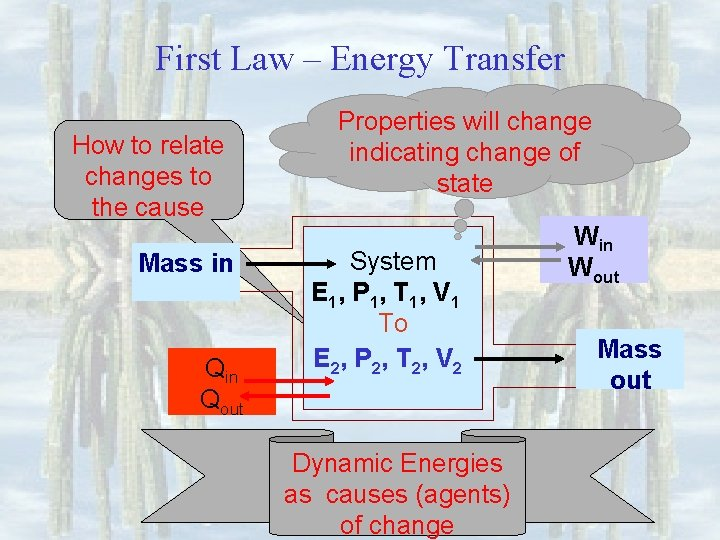First Law – Energy Transfer How to relate changes to the cause Mass in Qout Properties will change indicating change of state System E 1, P 1, T 1, V 1 To E 2, P 2, T 2, V 2 Dynamic Energies as causes (agents) of change Win Wout Mass out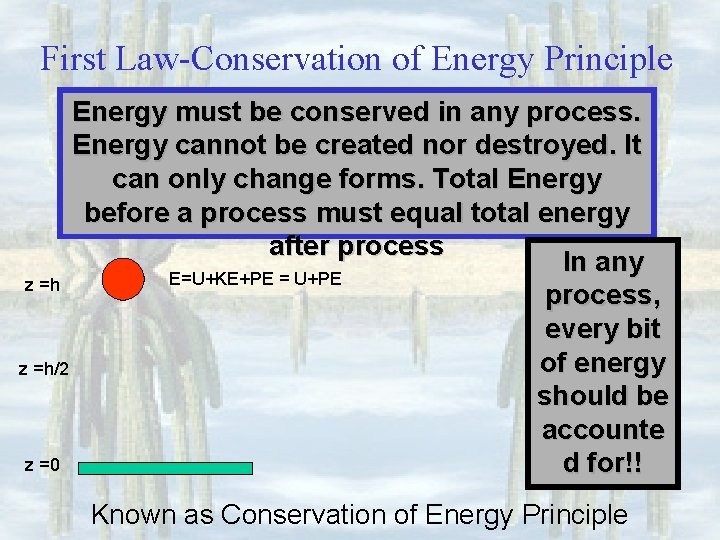First Law-Conservation of Energy Principle Energy must be conserved in any process. Energy cannot be created nor destroyed. It can only change forms. Total Energy before a process must equal total energy after process In any E=U+KE+PE = U+PE z =h process, every bit of energy z =h/2 should be accounte z =0 d for!! Known as Conservation of Energy Principle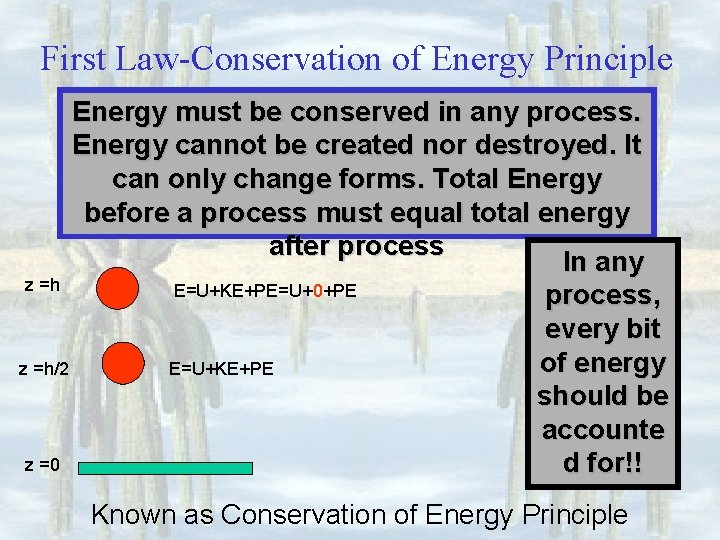First Law-Conservation of Energy Principle Energy must be conserved in any process. Energy cannot be created nor destroyed. It can only change forms. Total Energy before a process must equal total energy after process In any z =h E=U+KE+PE=U+0+PE process, every bit of energy z =h/2 E=U+KE+PE should be accounte z =0 d for!! Known as Conservation of Energy Principle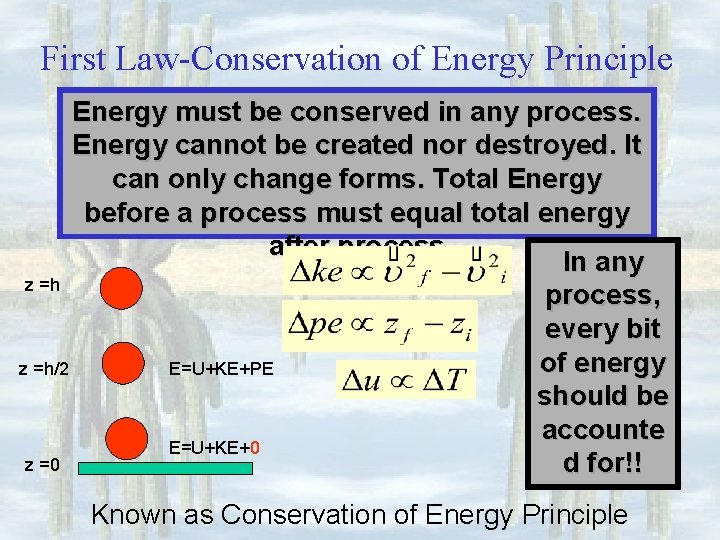First Law-Conservation of Energy Principle Energy must be conserved in any process. Energy cannot be created nor destroyed. It can only change forms. Total Energy before a process must equal total energy after process In any z =h process, every bit of energy z =h/2 E=U+KE+PE should be accounte E=U+KE+0 z =0 d for!! Known as Conservation of Energy Principle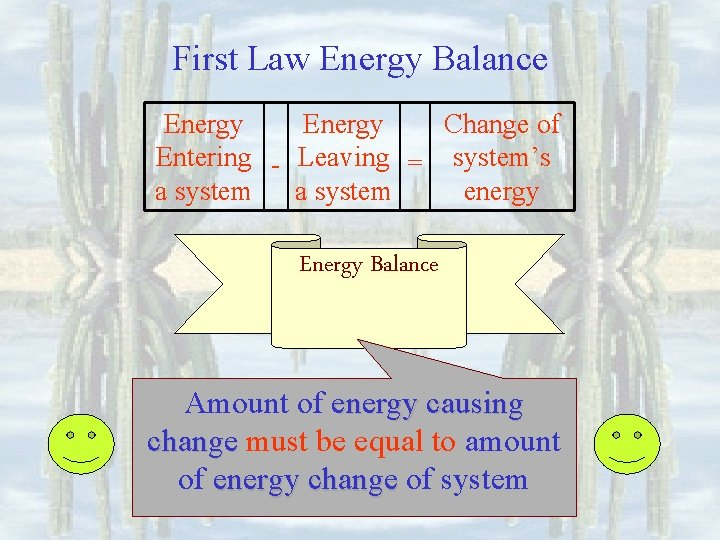First Law Energy Balance Energy Change of Entering - Leaving = system’s a system energy Energy Balance Amount of energy causing change must be equal to amount of energy change of system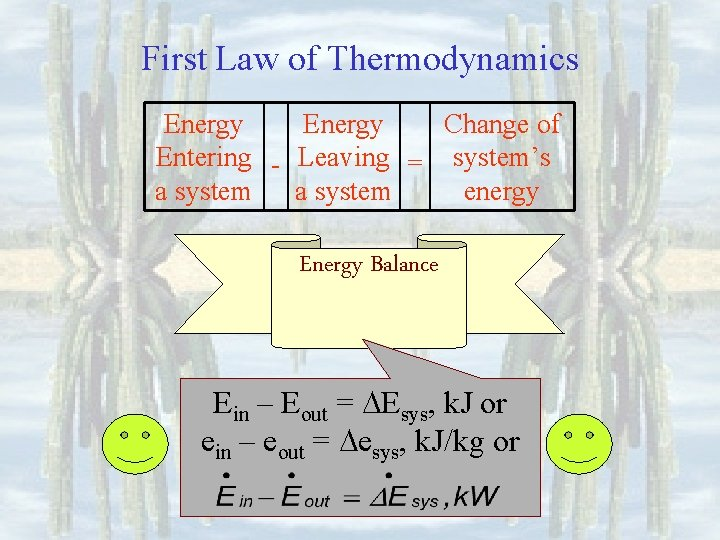First Law of Thermodynamics Energy Change of Entering - Leaving = system’s a system energy Energy Balance Ein – Eout = Esys, k. J or ein – eout = esys, k. J/kg orFirst Law of Thermodynamics How to relate changes to the cause Mass in Qout Properties will change indicating change of state System E 1, P 1, T 1, V 1 To E 2, P 2, T 2, V 2 Dynamic Energies as causes (agents) of change Win Wout Mass outCopyright © The Mc. Graw-Hill Companies, Inc. Permission required for reproduction or display. FIGURE 4– 7 The energy change of a system during a process is equal to the net work and heat transfer between the system and its surroundings. 4 -1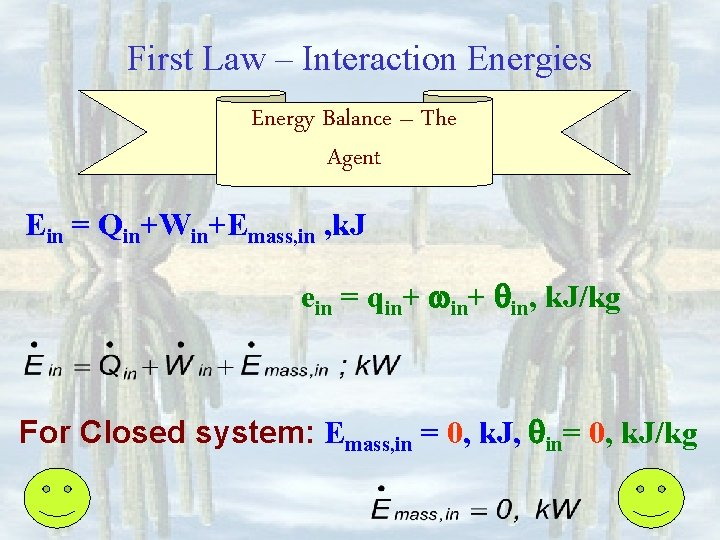First Law – Interaction Energies Energy Balance – The Agent Ein = Qin+Win+Emass, in , k. J ein = qin+ qin, k. J/kg For Closed system: Emass, in = 0, k. J, qin= 0, k. J/kg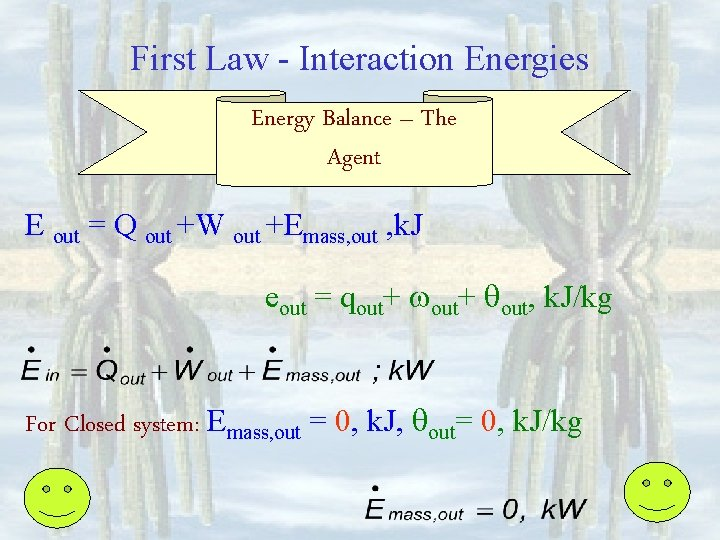First Law - Interaction Energies Energy Balance – The Agent E out = Q out +W out +Emass, out , k. J eout = qout+ qout, k. J/kg For Closed system: Emass, out = 0, k. J, qout= 0, k. J/kgFirst Law - System’s Energy Balance – The Change WIthin Energy change within the system, Esys = E 2 -E 1 is the sum of Internal energy change, U = U 2 – U 1 kinetic energy change, KE = KE 2 – KE 1 potential energy change, PE = PE 2 – PE 1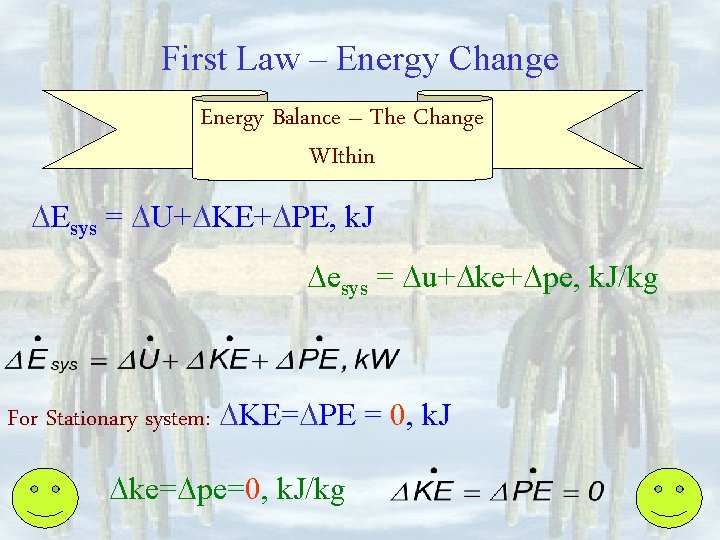First Law – Energy Change Energy Balance – The Change WIthin Esys = U+ KE+ PE, k. J esys = u+ ke+ pe, k. J/kg For Stationary system: KE= PE = 0, k. J ke= pe=0, k. J/kg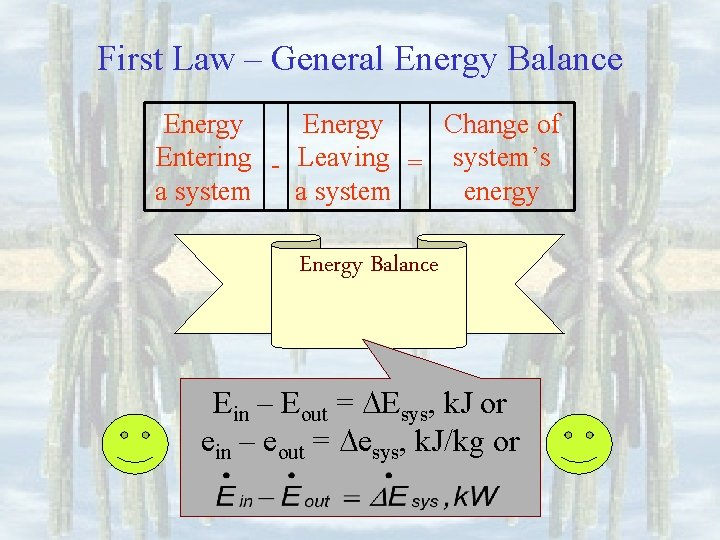First Law – General Energy Balance Energy Change of Entering - Leaving = system’s a system energy Energy Balance Ein – Eout = Esys, k. J or ein – eout = esys, k. J/kg or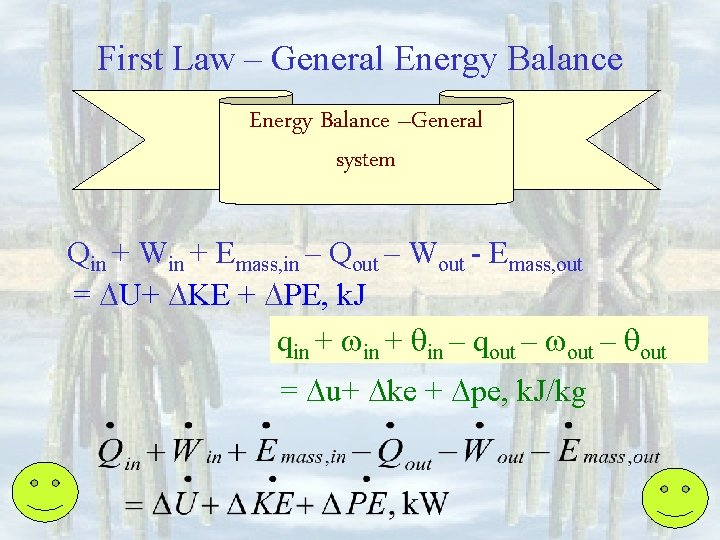First Law – General Energy Balance –General system Qin + Win + Emass, in – Qout – Wout - Emass, out = U+ KE + PE, k. J qin + qin – qout = u+ ke + pe, k. J/kgFirst Law – Stationary System Energy Balance –Stationary system Qin – Qout+ Win – Wout+ Emass, in - Emass, out = U+0+0 qin – qout+ in – out+ qin - qout = u + 0, k. J/kgFirst Law – Closed System Energy Balance –Closed system Qin – Qout+ Win – Wout+ 0 - 0 = U+ KE + PE, k. J qin – qout+ in – out + 0 – 0 = u+ ke + pe, k. J/kgFirst Law – Stationary & Closed Energy Balance –Stationary Closed system Qin – Qout+ Win – Wout+ 0 - 0 = U + 0 qin – qout+ in – out+ 0 - 0 = u + 0, k. J/kg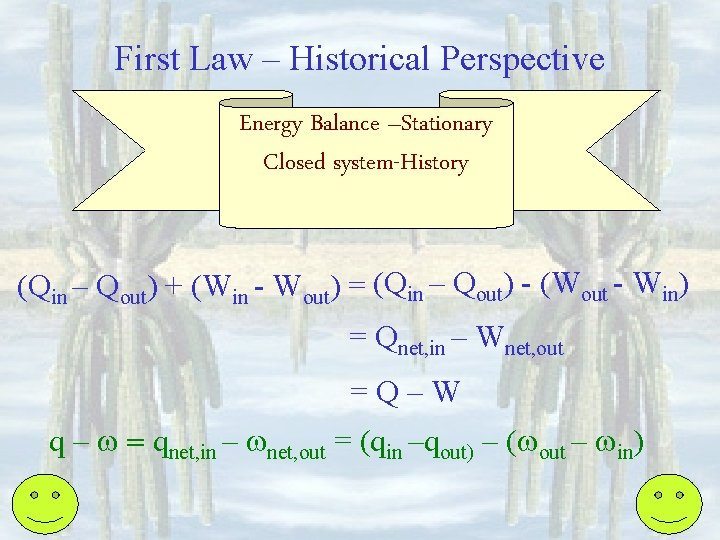First Law – Historical Perspective Energy Balance –Stationary Closed system-History (Qin – Qout) + (Win - Wout) = (Qin – Qout) - (Wout - Win) = Qnet, in – Wnet, out =Q–W q – = qnet, in – net, out = (qin –qout) – ( out – in)First Law – Adiabatic Process Energy Balance Stationary Closed system - Special Adiabatic: Adiabatic 0 – 0 + Win – Wout+ 0 - 0 = U + 0 0 – 0 + in – out+ 0 - 0 = u + 0, k. J/kg in = elec + pw + b, compress and out = b, expand k. J/kg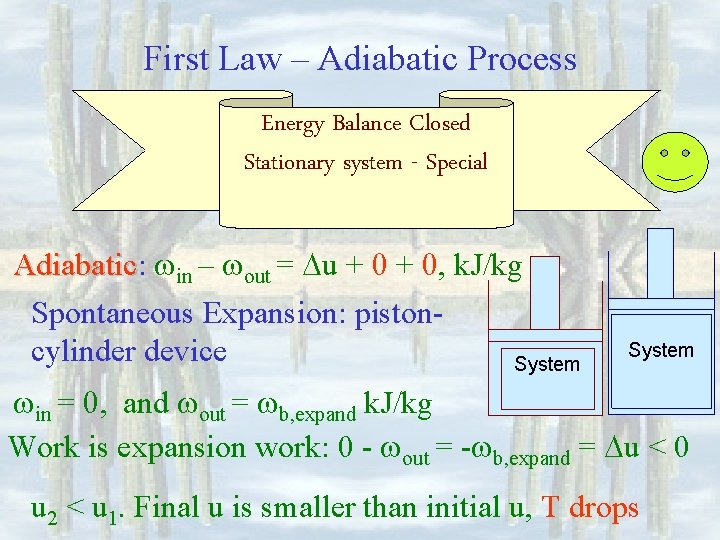First Law – Adiabatic Process Energy Balance Closed Stationary system - Special Adiabatic: Adiabatic in – out = u + 0, k. J/kg Spontaneous Expansion: pistoncylinder device System in = 0, and out = b, expand k. J/kg Work is expansion work: 0 - out = - b, expand = u < 0 u 2 < u 1. Final u is smaller than initial u, T dropsFirst Law – Adiabatic Process Energy Balance Closed Stationary system - Special Adiabatic: Adiabatic in – out - 0 = u+0+0, k. J/kg Compression: piston-cylinder device out = 0, and in = b, compress k. J/kg System Work is compression work: in = b, compress = u > 0 u 2 > u 1. Final u is bigger than initial u; T increasesFirst Law – Cyclic Process Energy Balance Closed Stationary system - Special Cyclic: Cyclic Qin – Qout+ Win – Wout+ 0 - 0 = Esys = 0 qin – qout+ in – out+ 0 - 0 = esys = 0, k. J/kg qin - qout = out – in or qnet, in = net, out Expansion: qin - 0 = out = b, expand All heat absorbed is used to do expansion work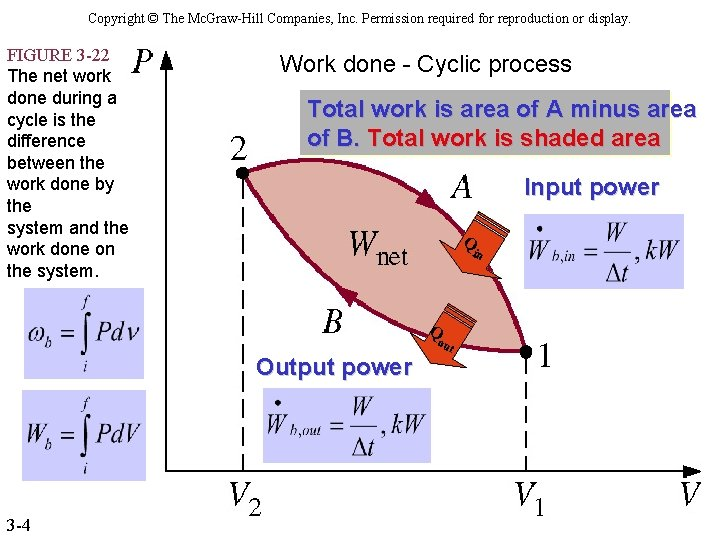Copyright © The Mc. Graw-Hill Companies, Inc. Permission required for reproduction or display. FIGURE 3 -22 The net work done during a cycle is the difference between the work done by the system and the work done on the system. Work done - Cyclic process Total work is area of A minus area of B. Total work is shaded area Input power Q in Q ou Output power 3 -4 t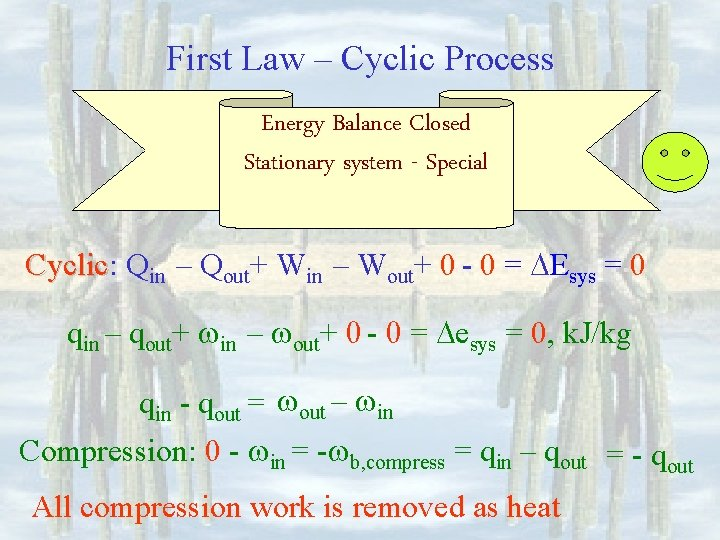First Law – Cyclic Process Energy Balance Closed Stationary system - Special Cyclic: Cyclic Qin – Qout+ Win – Wout+ 0 - 0 = Esys = 0 qin – qout+ in – out+ 0 - 0 = esys = 0, k. J/kg qin - qout = out – in Compression: 0 - in = - b, compress = qin – qout = - qout All compression work is removed as heat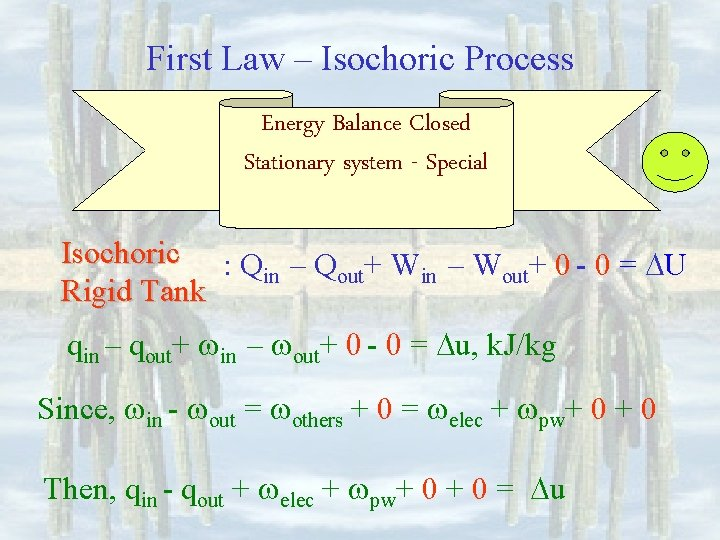First Law – Isochoric Process Energy Balance Closed Stationary system - Special Isochoric : Q – Q + W – W + 0 - 0 = U in out Rigid Tank qin – qout+ in – out+ 0 - 0 = u, k. J/kg Since, in - out = others + 0 = elec + pw+ 0 Then, qin - qout + elec + pw+ 0 = u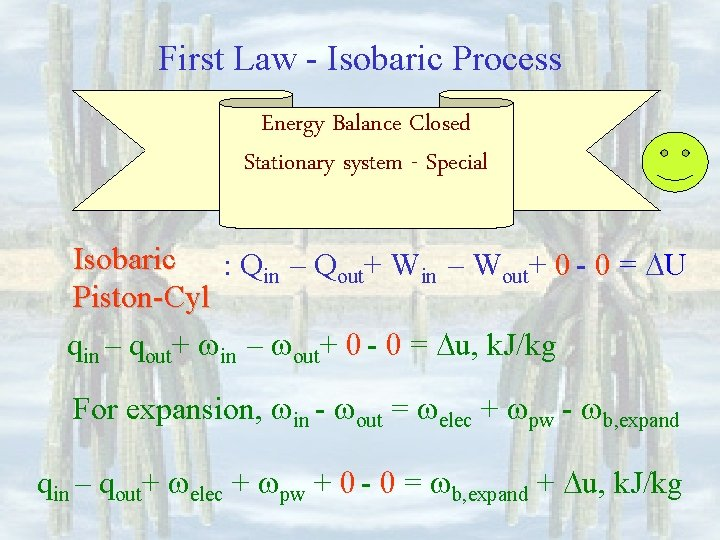First Law - Isobaric Process Energy Balance Closed Stationary system - Special Isobaric : Qin – Qout+ Win – Wout+ 0 - 0 = U Piston-Cyl qin – qout+ in – out+ 0 - 0 = u, k. J/kg For expansion, in - out = elec + pw - b, expand qin – qout+ elec + pw + 0 - 0 = b, expand + u, k. J/kg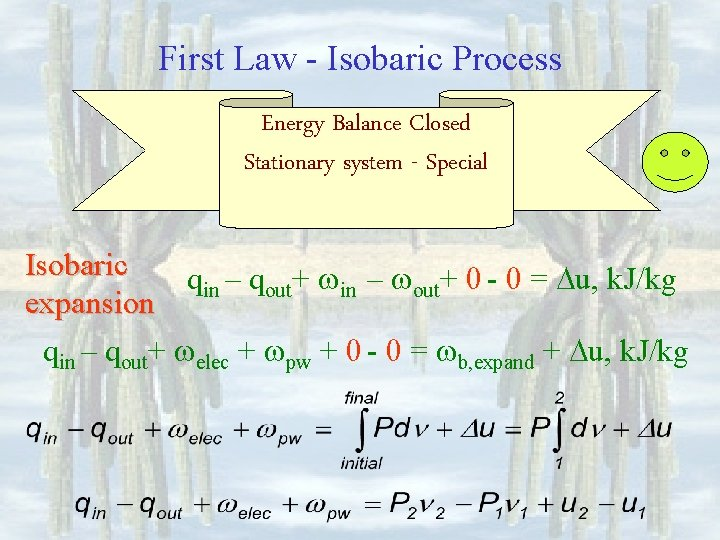First Law - Isobaric Process Energy Balance Closed Stationary system - Special Isobaric qin – qout+ in – out+ 0 - 0 = u, k. J/kg expansion qin – qout+ elec + pw + 0 - 0 = b, expand + u, k. J/kg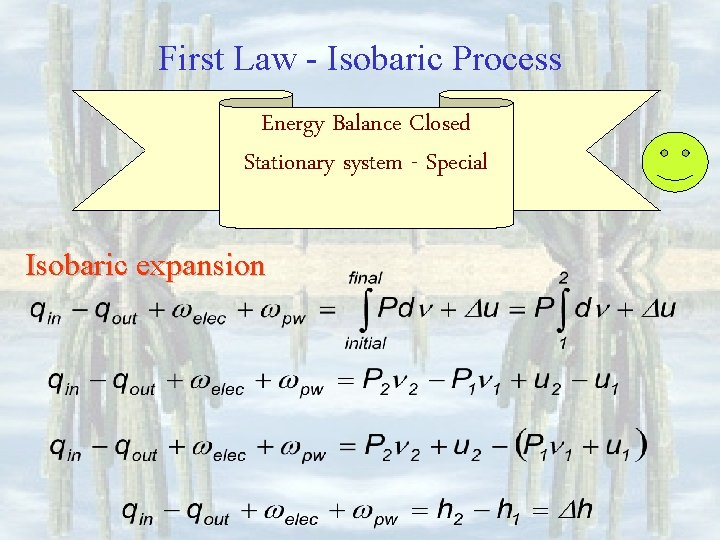First Law - Isobaric Process Energy Balance Closed Stationary system - Special Isobaric expansion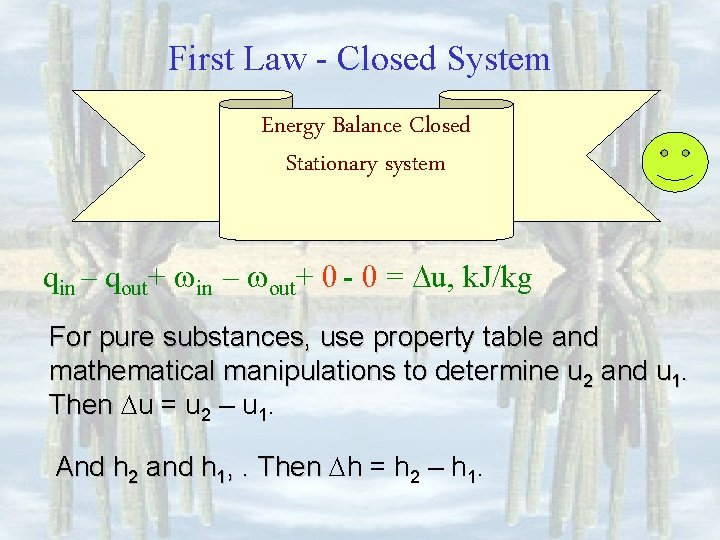First Law - Closed System Energy Balance Closed Stationary system qin – qout+ in – out+ 0 - 0 = u, k. J/kg For pure substances, use property table and mathematical manipulations to determine u 2 and u 1. Then u = u 2 – u 1. And h 2 and h 1, . Then h = h 2 – h 1.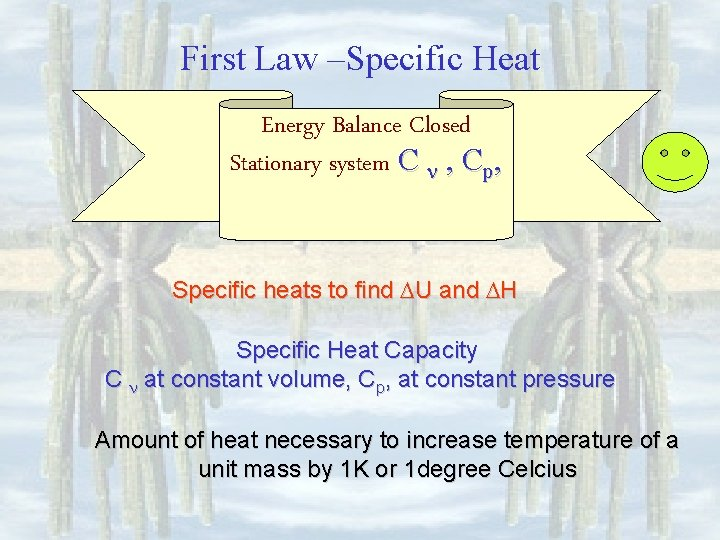First Law –Specific Heat Energy Balance Closed Stationary system C , Cp, Specific heats to find U and H Specific Heat Capacity C at constant volume, Cp, at constant pressure Amount of heat necessary to increase temperature of a unit mass by 1 K or 1 degree Celcius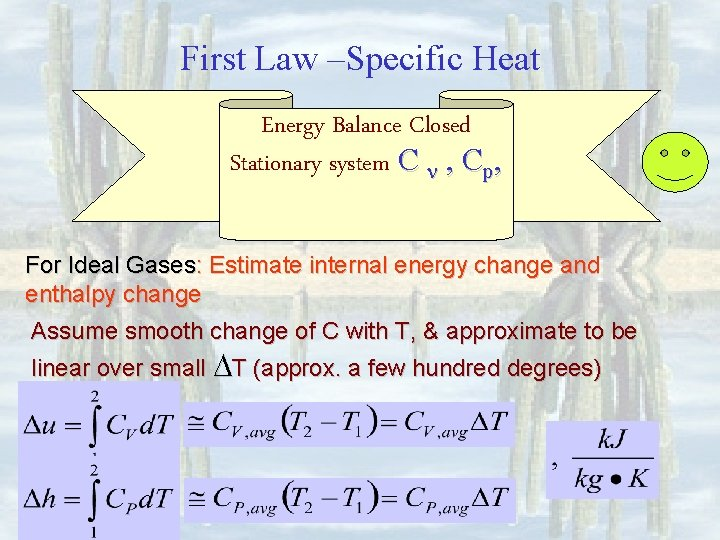First Law –Specific Heat Energy Balance Closed Stationary system C , Cp, For Ideal Gases: Estimate internal energy change and enthalpy change Assume smooth change of C with T, & approximate to be linear over small T (approx. a few hundred degrees)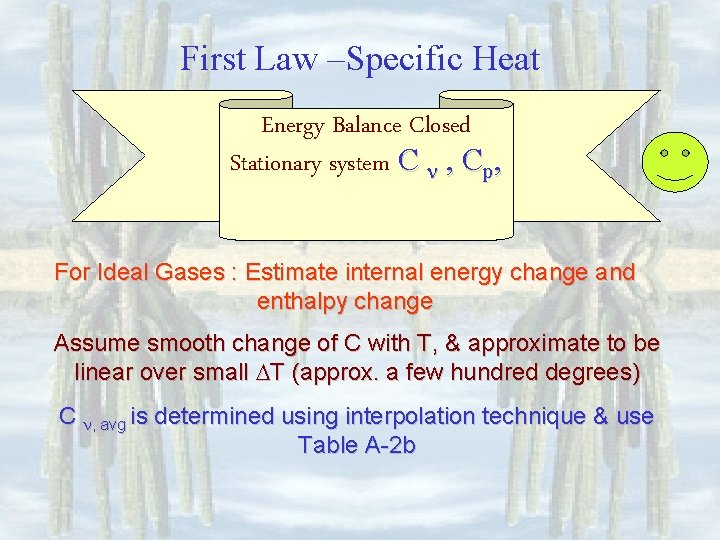First Law –Specific Heat Energy Balance Closed Stationary system C , Cp, For Ideal Gases : Estimate internal energy change and enthalpy change Assume smooth change of C with T, & approximate to be linear over small T (approx. a few hundred degrees) C , avg is determined using interpolation technique & use Table A-2 b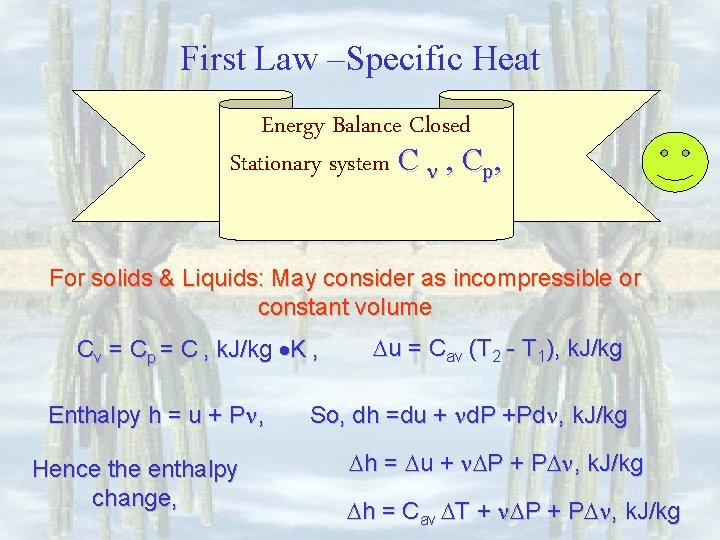First Law –Specific Heat Energy Balance Closed Stationary system C , Cp, For solids & Liquids: May consider as incompressible or constant volume Cv = Cp = C , k. J/kg K , Enthalpy h = u + P , Hence the enthalpy change, u = Cav (T 2 - T 1), k. J/kg So, dh =du + d. P +Pd , k. J/kg h = u + P + P , k. J/kg h = Cav T + P + P , k. J/kg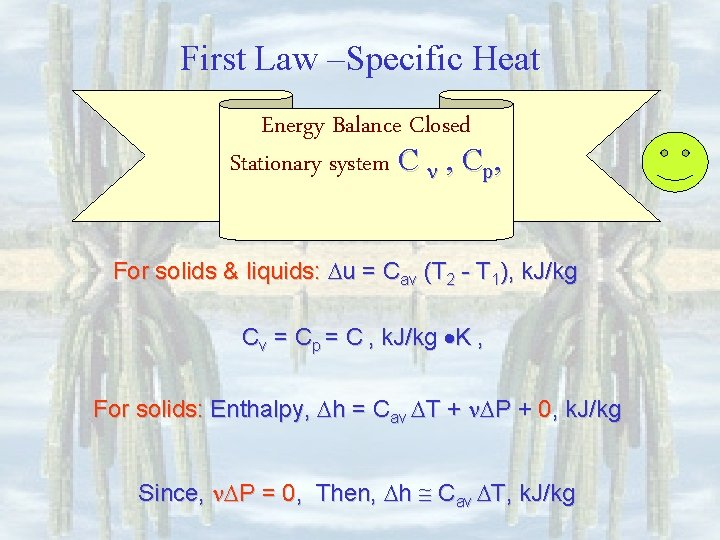First Law –Specific Heat Energy Balance Closed Stationary system C , Cp, For solids & liquids: u = Cav (T 2 - T 1), k. J/kg Cv = Cp = C , k. J/kg K , For solids: Enthalpy, h = Cav T + P + 0, k. J/kg Since, P = 0, Then, h Cav T, k. J/kg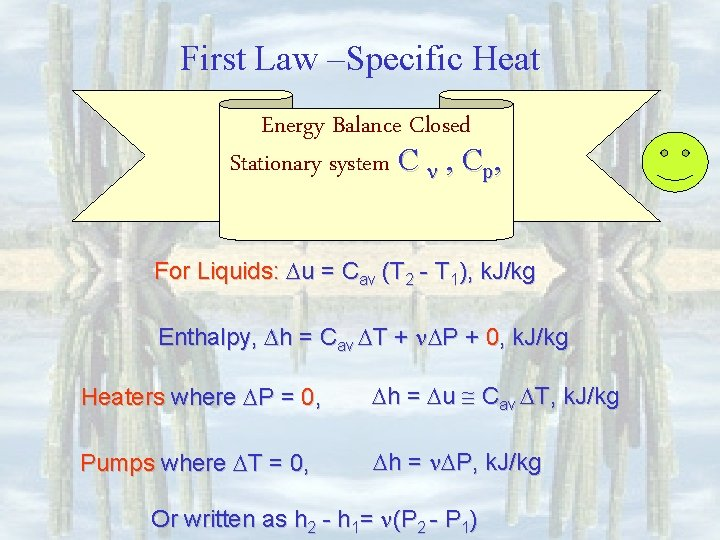First Law –Specific Heat Energy Balance Closed Stationary system C , Cp, For Liquids: u = Cav (T 2 - T 1), k. J/kg Enthalpy, h = Cav T + P + 0, k. J/kg Heaters where P = 0, h = u Cav T, k. J/kg Pumps where T = 0, h = P, k. J/kg Or written as h 2 - h 1= (P 2 - P 1)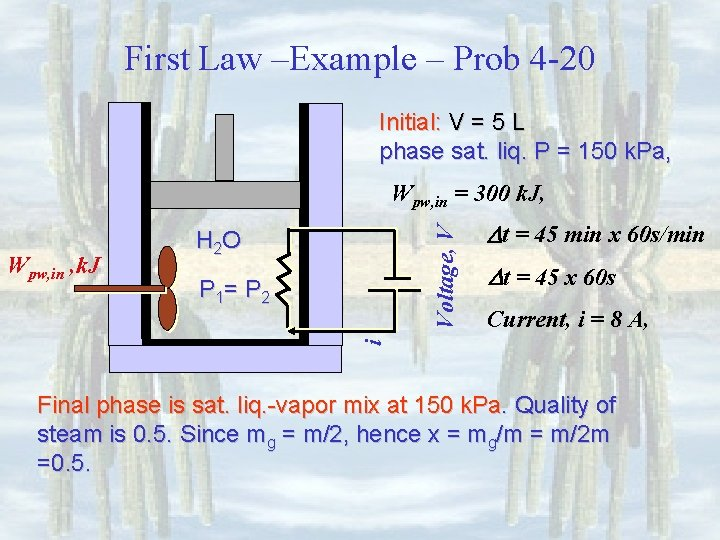First Law –Example – Prob 4 -20 Initial: V = 5 L phase sat. liq. P = 150 k. Pa, H 2 O P 1= P 2 t = 45 min x 60 s/min t = 45 x 60 s Current, i = 8 A, i Wpw, in , k. J Voltage, V Wpw, in = 300 k. J, Final phase is sat. liq. -vapor mix at 150 k. Pa. Quality of steam is 0. 5. Since mg = m/2, hence x = mg/m = m/2 m =0. 5.First Law –Example – Prob 4 -20 Initial: V = 5 L phase sat. liq. P = 150 k. Pa, Wpw, in , k. J Voltage, V Wpw, in = 300 k. J, H 2 O t = 45 x 60 s Current, i = 8 A, i P 1= P 2 t = 45 min x 60 s/min 1= [email protected] k. Pa, = 0. 0010528 m 3/kg x 2 = 0. 5 h 1= [email protected] k. Pa, = 467. 1 k. J/kg 2= [ f + x 2 vfg]@150 k. Pa, = 0. 579938 m 3/kg h 2= [hf + xhfg]@150 k. Pa =1580 k. J/kgFirst Law –Example – Prob 4 -20 Energy balance, Ein - Eout = Esys 0 + Win + 0 – Wout - 0 = U+ 0 + 0, k. J Wpw, in + We, in= U + Wout Then where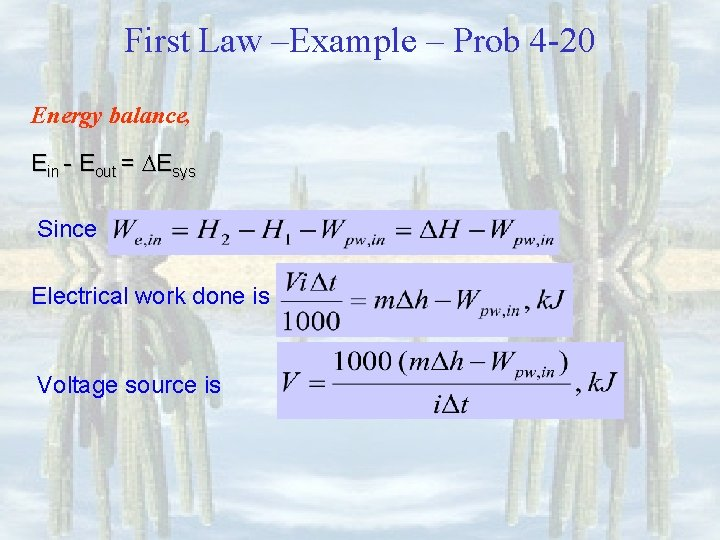First Law –Example – Prob 4 -20 Energy balance, Ein - Eout = Esys Since Electrical work done is Voltage source isFirst Law –Example – Prob 4 -20 Energy balance, Ein - Eout = Esys Voltage source is Note that the unit k. J/s = Volts-Ampere or VA